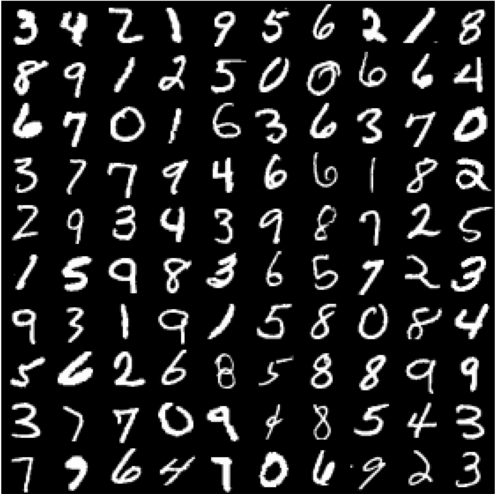# Build the MNIST model with your own handwritten digits using TensorFlow, Keras, and PythonThis post will give you an idea about how to use your own handwritten digits images with Keras MNIST dataset.

If you don’t know how to build a model with MNIST data please read my previous article.

Recently one guy contacted me with a problem by saying that his trained model or my trained model is giving trouble in recognizing his handwritten digits. I tried to help him at the same time I tested my model too. I am able to replicate the issue.

It looks like overfit issue and also the images in Keras dataset are in center position so model trained like that. So I thought let’s tackle the problem by giving my own handwritten images.

In this process, I also realized giving one image doesn’t help in training we need to give a good number of images so that it can learn from them.

To do this we can use existing training model and train a new model using it through transfer learning but I am new to this section so I am going to try that later, for now, I tweaked my existing code a little to complete this job.

Without wasting much time Let’s get into the code

Let’s import the packages required to do this task

```from keras.datasets import mnist
from keras.models import Sequential
from keras.layers import Dense
from keras.layers import Dropout
from keras.layers import Flatten
from keras.layers.convolutional import Conv2D
from keras.layers.convolutional import MaxPooling2D
from keras.utils import np_utils
from PIL import Image
import numpy as np
import os```

Let’s load the Keras MNIST dataset first

```# load data
(X_train, y_train), (X_test, y_test) = mnist.load_data()```

Now let’s reshape the data according to CNN expectations

```# Reshaping to format which CNN expects (batch, height, width, channels)
X_train = X_train.reshape(X_train.shape, X_train.shape, X_train.shape, 1).astype('float32')
X_test = X_test.reshape(X_test.shape, X_test.shape, X_test.shape, 1).astype('float32')```

It’s time for adding our own images to training and test data. For that below function will help you

```# To load images to features and labels
list_of_files = os.listdir(image_directory)
for file in list_of_files:
image_file_name = os.path.join(image_directory, file)
if ".png" in image_file_name:
img = Image.open(image_file_name).convert("L")
img = np.resize(img, (28,28,1))
im2arr = np.array(img)
im2arr = im2arr.reshape(1,28,28,1)
features_data = np.append(features_data, im2arr, axis=0)
label_data = np.append(label_data, [image_label], axis=0)
return features_data, label_data```

Basically, this function takes image label, image directory, features data, labels data as input.
It lists all files present in image directory and then checks whether it is png file or not(if you have jpg images then change the “.png” condition to “.jpg”).
Then loads the image and convert that to an array which is similar to
features data and adds the image array to it.
Takes image label and add that to label_data.
Once it adds all our images in that folder/directory to current dataset returns them back.

Now let’s give our own images directories to load them to existing dataset.

```# Load your own images to training and test data
X_train, y_train = load_images_to_data('1', 'data/mnist_data/train/1', X_train, y_train)
X_test, y_test = load_images_to_data('1', 'data/mnist_data/validation/1', X_test, y_test)```

Let’s normalize the data now.

```# normalize inputs from 0-255 to 0-1
X_train/=255
X_test/=255```

We have labels but those are not categorized. So let’s do it now.

```# one hot encode
number_of_classes = 10
y_train = np_utils.to_categorical(y_train, number_of_classes)
y_test = np_utils.to_categorical(y_test, number_of_classes)```

It’s time to create our model

```# create model
model = Sequential()
model.add(Conv2D(32, (5, 5), input_shape=(X_train.shape, X_train.shape, 1), activation='relu'))

Added Dropouts to make sure we don’t overfit the model

Let’s compile our model

```# Compile model

It’s time to train the model

```# Fit the model
model.fit(X_train, y_train, validation_data=(X_test, y_test), epochs=7, batch_size=200)```

Kept 7 epochs because after 7th I didn’t see much accuracy improvements.

Our model is ready just to make sure whether our model trained properly or not let’s load an image in validation folder and check our model is performing well or not

```img = Image.open('data/mnist_data/validation/1/1_2.png').convert("L")
img = np.resize(img, (28,28,1))
im2arr = np.array(img)
im2arr = im2arr.reshape(1,28,28,1)
y_pred = model.predict_classes(im2arr)
print(y_pred)```

I got the output as

``

Congrats!!! Our model is trained with our own images too.

You will find this code(notebook) here.

Peace. Happy Coding.

## 6 thoughts on “Build the MNIST model with your own handwritten digits using TensorFlow, Keras, and Python”

1.Khai Hong says:

Hi,

img = Image.open(‘data/mnist_data/validation/1/1_2.png’).convert(“L”)
img = np.resize(img, (28,28,1))
im2arr = np.array(img)
im2arr = im2arr.reshape(1,28,28,1)

I tried this but I get all 0 for my image array, could you please advise?

1.tankala says:

Hi Khai Hong,

What accuracy you got?
Is it giving like that for this image or for every image?

2.Haval Sadeq says:

Hi,

Thanks a lot for the blog,
The code worked successfully and gave the correct result.
I have seen that you integrated personal dataset with MNIST.
Could you explain how it is possible to use my own dataset for training and testing without using MNIST dataset?

Thank you.

1.tankala says:

I am glad you liked my blog. Skip below code part.
(X_train, y_train), (X_test, y_test) = mnist.load_data()

Need to write a bit of code around creating these variables then you can use your own dataset

3.Luke says:

Hi,
Found your blog a few days ago and I have been enjoying coding along!
When running the last code the prediction always outputs “1” no matter which image file I enter and was hoping you could help.

Thank you

1.Luke says:

Hi again,
Would you be able to tell me the structure of your directories?